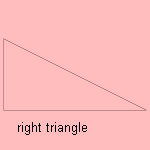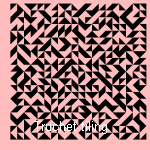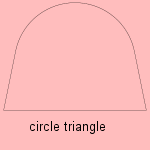# triangle

## line

The triangle has been studied in trigonometry by many scholars, already in the Ancient Greek times.
It is also called a trigon.

The triangle is the only polygon for which the shape remains the same, as long as the length of the sides doesn't change (a rafter).

The triangle is a simple figure, but however, many triangles can be distinguished, many of them carrying an own name. For the acute-angled triangle (or acute triangle) all angles are smaller than 90 degrees. When a triangle has an obtuse angle, it's an obtuse-angled triangle (or obtuse triangle).

The triangle curve repeats the isosceles triangle, a triangle with two equal legs.A right-angled triangle (or right triangle) has one rectangular angle. Then, Pythagoras' theorem states the relation between the oblique side a and the other two sides as a2 = b2 + c2.
The triangle inequality relates the three sides in a triangle: a < b + c.

The saw-tooth curve repeats the right triangle. For equal sides of the right angle we've got of the right . This triangle is used in mathematical lessons as 'triangle' or 'set square'. Sometimes this set square has the form of a special right triangle, a right triangle with angles of 30 and 60 degrees.In 1704, the Dominican priest Sébastien Truchet (1657-1729) published a text 3) in which he showed that an infinite number of motifs can be used to generate a pattern.
He considered the possible patterns formed by tilings of (filled) right isosceles triangles oriented at the four corners of a square: the Truchet tiling. There are four possible orientations that can be combined, making beautiful patterns.

The characteristic triangle is the triangle with infinitesimal sides dx, dy and ds. This 'triangulum characteristicum' has been used in the 17th century by Pascal, Snellius and Leibniz, while developing infinitesimal mathematics. It seems that is was Leibniz who introduced this expression.In a equilateral triangle (or regular triangle) the three sides have equal length. These triangles can fill the plane 1). This quality has already been found by Pythagoras (500 BC). The group of scholars around Pythagoras had a special liking for numbers, which had for them a magical meaning. They studied the 'figure numbers' (or 'polygon numbers'), constructed from a regular polygon.
In the following way, from a regular triangle:and so on.
This results in the triangular numbers 1, 3, 6, 10, 15, and so on.
In Pascal's triangle we find these same numbers as binomial coefficients (n over k) 2):
1
1    1
1    2    1
1    3    3     1
1    4    6     4     1
1    5    10    10     5      1

These coefficients are often used in probability theory and also in formulae for the nth power and the nth derivative. In probability theory the triangle diagram, is used, a way to express the a function of three variables, in a triangle grid.

A curve that can be turned continuously inside an equilateral triangle, is called a delta curve. There are an infinite number of these curves.

The Dutch drawer Escher was responsible for the 'impossible triangle': an impossible (perspective) triangle that can be drawn, however.
When coping with a project, there is a tension between time, money and quality. You can show this graphically: when you try to diminish the amount of time spent, that cost you money or quality. This is called the devil's triangle.

What triangular objects we see around us? When going to a symphony orchestra we hear the triangle, visiting the sea we can find the triangle mussel the triangle shell and the triangle crab. We have also triangular parts ourselves:

• the 'triangular nerve' (the fifth cerebral nerve)
• the 'triangular bone': a bone in the back row of carpal bones
• the 'triangular muscle': the muscle that goes from the clavicle, shoulder and shoulder-blade to the outer side of the upper arm

A small pair of trunks or pants is called a triangle (in the Netherlands).

It is rather popular to use the triangular form of geographic regions in their names:

• the 'Bermuda triangle' - a sea east of the U.S. that is notorious for his mysterious disappearances, in apparent good weather conditions 3)
• in the 'gold triangle' is the world's largest resource of opium: the borderland of Thailand, Burma with Laos; the sea-area between Costa Rica, Colombia and Ecuador is also denoted as a 'golden triangle'.
• the 'black triangle' or triangle of death is the extremely polluted borderland of Poland, Czechia with Germany
• the 'iron triangle' is the area northwest of Saigon, where the Vietnam war struck heavy wounds
• the 'brown triangle' is the region of Munich, Nuremberg and Berchtesgarden in Germany, bastion of the brown-clothed nazis
• the 'dark triangle' was a region in Canada, where in 1997 ice-rain made collapse electricity pylons for 3 million people
• 'The Triangle' is North Carolina's version of Silicon Valley
• in Congo a large area of rainforest is called the Goualogo Triangle

Measuring land for mapping by use of trigonometry is called triangulation.

In the 17th and 18th century traders of the Dutch Republic made 'triangular journeys'. Goods were transported by ship in three laps. At first Dutch goods as brandy  were transported to western Africa. This was exchanged for slaves who were transported to the Western Indies. There the slaves were exchanged for goods as cacao, cotton and tobacco, to be brought home. These journeys took about 15 months each.
The triangle manifests itself also in social behavior: one speaks of the eternal triangle when there exist intimate relations between three persons. In the Netherlands the regional cooperation of the Government with the police and the Counsel for the Prosecution is called the 'regional triangle'.

The (mostly regular) triangle is used as a symbol for certain groups or organizations:

• the pink triangle is the symbol for the homosexual movement; its form has been derived from the German concentration camps, where homosexuals were forced to wear those triangles.
• the red triangle is the symbol of the YMCA
• the blue triangle is the symbol of the YWCA

In traffic a triangular sign has always a warning function (a square sign gives a remark). The warning triangle is also used when your car has a break-down.

Lifting a triangle in 3d makes a pyramid.

At last we know the triangles, for which its quality depends on its position regarding another triangle. The cyclic triangle, the inscribed triangle, the circumscribed triangle and the tangent triangle did we already see at the polygon section.

Other special triangles are:

• the pedal triangle is formed by the dropped altitudes in a triangle
• the first Brocard's triangle and the second Brocard's triangle are rather complex triangles, invented by the 19th century French mathematician Brocard
• perspective triangles: two triangles A1B1C1 and A2B2C2 are perspective in relation to each other when the lines A1A2, B1B2 en C1C2 meet each other in one point. It was Desargues who formulated the theory of these phenomena (1648).A series of triangles can be used to form a spiral of Theodore of Cyrene that approximates the logarithmic spiral.

My 9 year old son Jelle showed me the circle triangle, a combination of a part of a circle and a triangle.

For a triangle inscribed in a circle with respect to a point P on the circle a so called Simson line can be constructed. The envelope of these Simson lines is the deltoid.

Three tangent circles can fill a triangle as the Malfatti circles.

notes

1) When a reflection is admitted, this holds for every triangle.

2) In Pascal's 'Triangle aritmetique', published in 1764 (after Pascal's death), the relation between the binomial coefficients and Pascal's triangle is shown.

3) Some investigators doubt the mysterious character: it should be bad weather at time of those accidents, though.